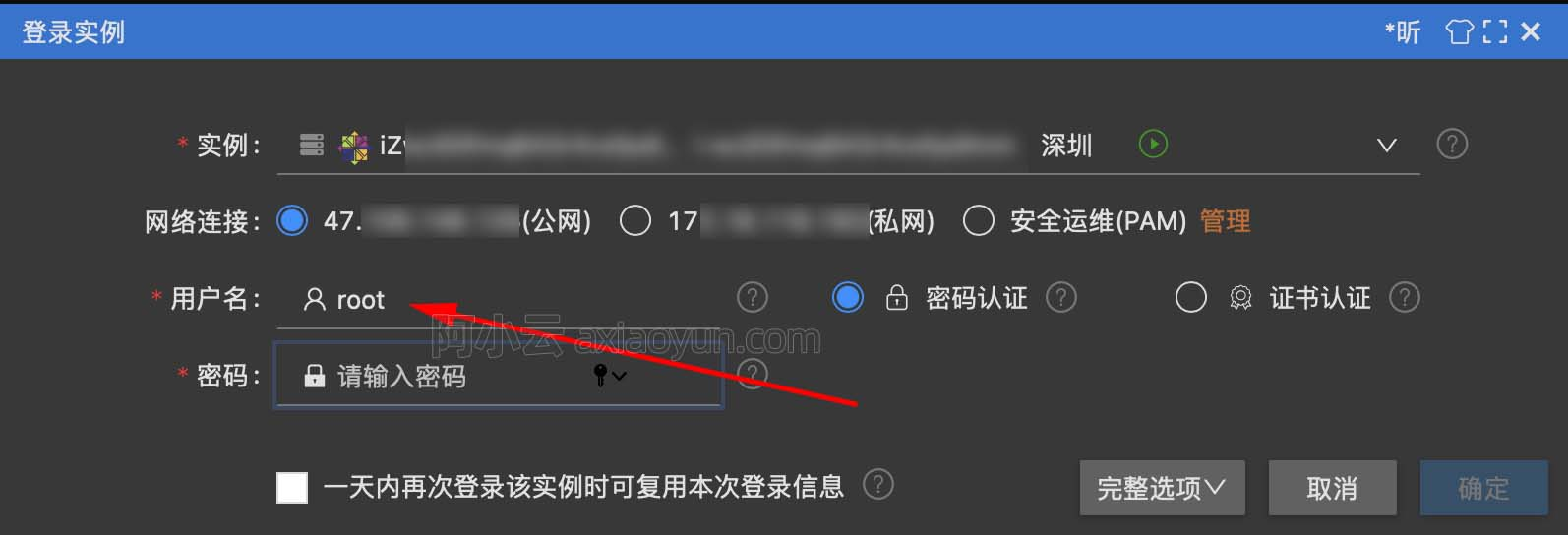+关注继续查看

练习3-77

原文

Exercise 3.77. The integral procedure used above was analogous to the “implicit” definition of the infinite stream of integers in section 3.5.2. Alternatively, we can give a definition of integral that is more like integers-starting-from (also in section 3.5.2):

(define (integral integrand initial-value dt)
(cons-stream initial-value
(if (stream-null? integrand)
the-empty-stream
(integral (stream-cdr integrand)
(+ (* dt (stream-car integrand))
initial-value)
dt))))

When used in systems with loops, this procedure has the same problem as does our original version of integral. Modify the procedure so that it expects the integrand as a delayed argument and hence can be used in the solve procedure shown above.

代码

(define (integral delayed-integrand initial-value dt)
(cons-stream initial-value
(let ((integrand (force delayed-integrand)))
(if (stream-null? integrand)
the-empty-stream
(integral (delay (stream-cdr integrand))
(+ (* dt (stream-car integrand))
initial-value)
dt)))))

http://blog.csdn.net/nomasp26770 010004 014462 02496 010880 0windows server 2008阿里云ECS服务器安全设置

9146 07455 022357 04470 07327 0
542

0

《零基础CSS入门教程》

《零基础HTML入门教程》

《2021云上架构与运维峰会演讲合集》